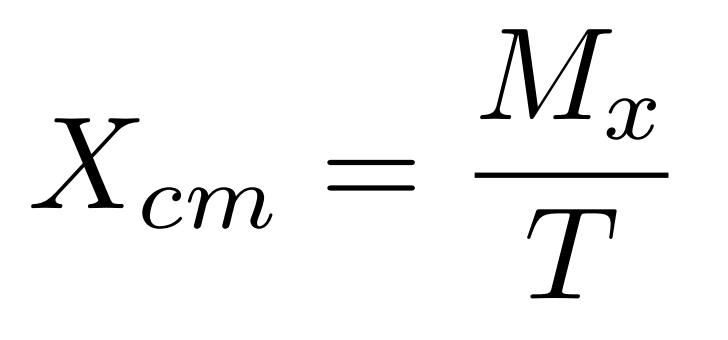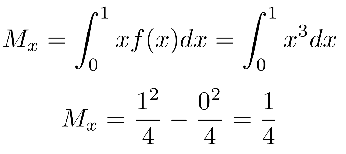# Center of Mass (Calculus)

The center of mass (CM) is a weighted average that represents the mean (average) position in a given system.

Have you ever tried to balance a pencil on your finger? The point at which the pen rests on your finger successfully would be its center of mass. The lower an object’s CM, the more stable it will be under motion. That’s why unicycles are challenging to balance, but a boat with a heavy keel remains stable during even the strongest of storms.

## Center of Mass Definition: One Dimension

The definition is based on the following formula:Where:

• Xcm is the center of mass,
• Mx is the moment, and
• T is the total mass.

In calculus, the moment can be written as the following integral:

Mx = x f(x) dx

and the total mass can be written in similar terms:

T= f(x) dx

## Example: One Dimension

Example Problem: A canoe has a density of f(x) = x2, where 0 ≤ x ≤ 1. Where is the center of mass for the canoe?

Solution: To find the CM for this system, let’s first see the momentNext, let’s calculate the total massTherefore, the center of mass is atThis result should make sense, intuitively. Most of the weight would be towards the x = 1 end of the canoe since the density is higher there.

## Definition: Two Dimensions

Sometimes, you may be asked to evaluate the center of mass for a two-dimensional object. The same mathematics that applies to the one-dimensional case can be extended to the two-dimensional case.

## Example: Two Dimensions

Problem: Assume that a thin, triangular plate of uniform density is bounded by the x-axis, y-axis, and the function f(x) = 1 – x. Find the center of mass of the plate.

Solution: We can follow the same procedure for the x CM as we did in the one-dimensional problem.andSo, the center of mass for the triangular plate is at (⅓, ⅓). If you had a real triangular plate, it could be balanced at that point.

## Summary

Ultimately, the center of mass for any object or system will be equal to its moment divided by the total mass for each dimension. The center of mass represents the system’s “average” value–the point at which the object or system is centered.

CITE THIS AS:
Stephanie Glen. "Center of Mass (Calculus)" From StatisticsHowTo.com: Elementary Statistics for the rest of us! https://www.statisticshowto.com/center-of-mass-calculus/